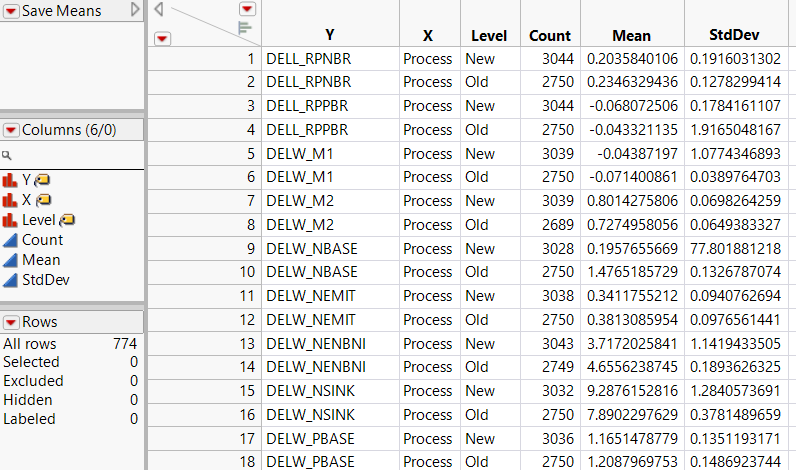Publication date: 05/24/2021

## Means Data Table

The Means data table contains a row for each combination of response and X level. For the Probe.jmp sample data table, there are 387 response variables, each tested against Process at two levels. The Means table contains 387x2 = 774 rows.

Figure 22.6 Means Data TableThe Means data table includes the following columns:

Y

The continuous response variables.

X

The categorical variables.

Level

The level of the categorical X variable.

Count

The count of values in the corresponding Level.

Mean

The mean of the Y variable for the specified Level.

StdDev

The standard deviation of the Y variable for the specified Level.

Robust Mean

The robust M-estimate of the mean. Appears when you select the Robust option on the launch window.